Function Repository Resource:

# ProjectGraphics3D

Project 3D graphics onto a plane

Contributed by: Wolfram Staff (original content by Alfred Gray)
 ResourceFunction["ProjectGraphics3D"][graphics3D,p] projects an image of graphics3D onto a plane through point p and perpendicular to the line from the center of the graphics3D to p. ResourceFunction["ProjectGraphics3D"][graphics3D,{e1,e2},p] projects an image of graphics3D onto a plane with basis vectors {e1, e2} at p, along the line from the origin to p. ResourceFunction["ProjectGraphics3D"][graphics3D,{e1,e2},p,center] projects along the line from center to p, as seen from Infinity.

## Details and Options

The center of the graphic is computed using PlotRange.
ResourceFunction["ProjectGraphics3D"] takes the same options as Graphics3D plus PlotStyle.

## Examples

### Basic Examples (3)

Project a curve onto a plane:

 In:=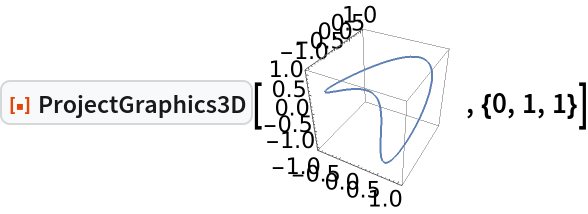Out=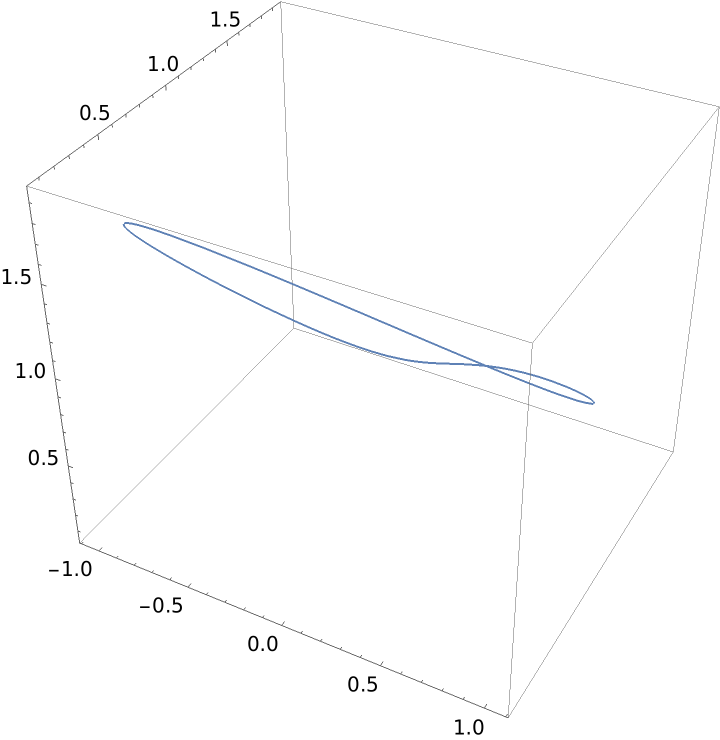Project a polyhedron:

 In:=Out=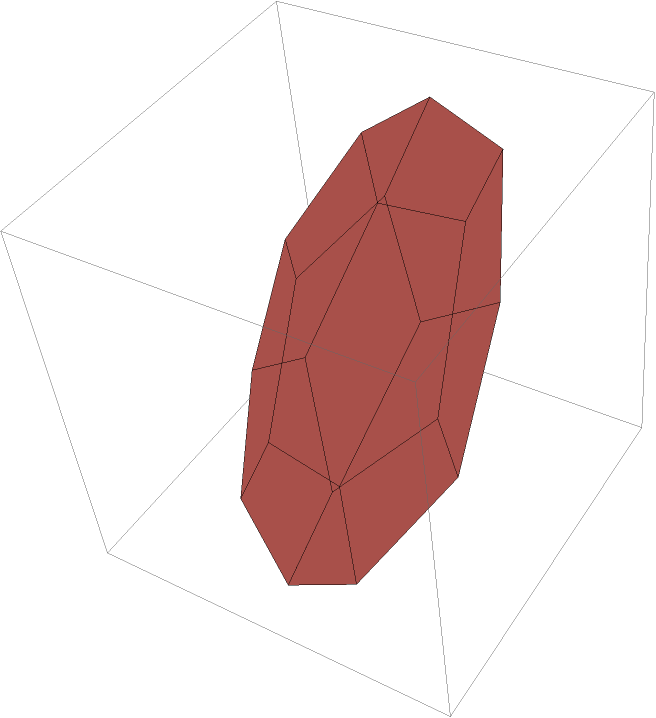Project a surface:

 In:=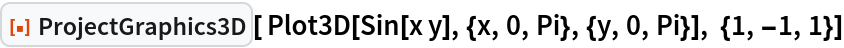Out=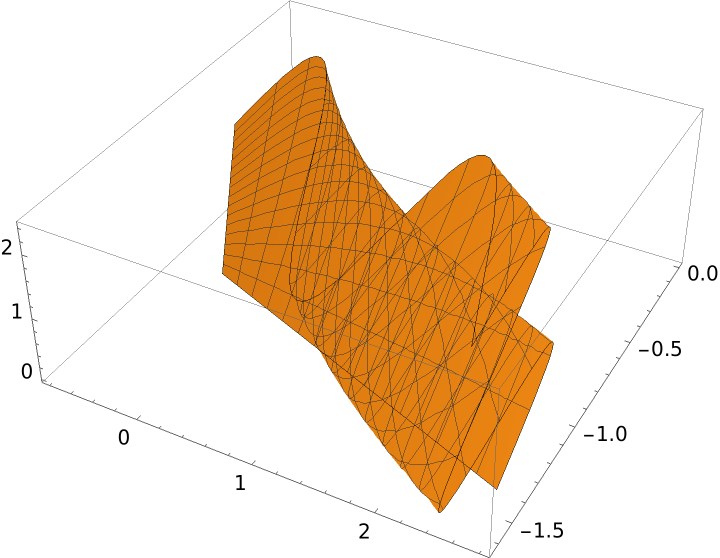### Scope (3)

A curve not defined at some ranges:

 In:=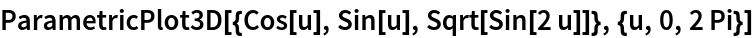Out=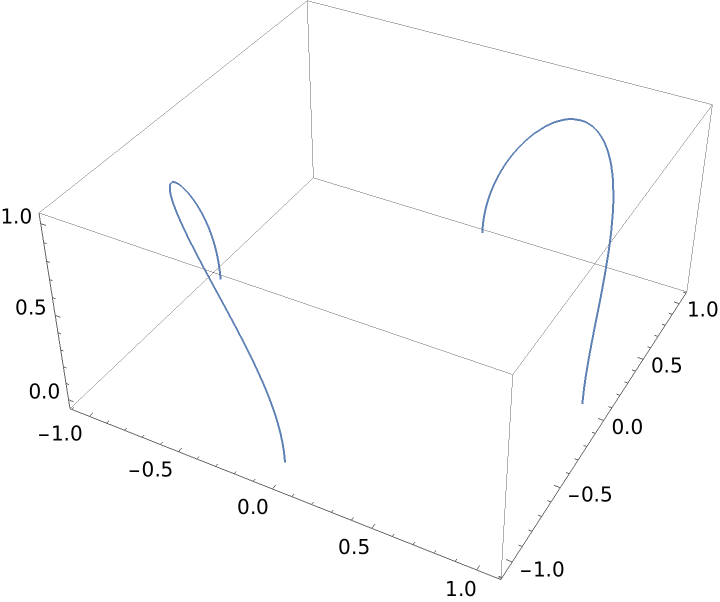Show it with a projection:

 In:=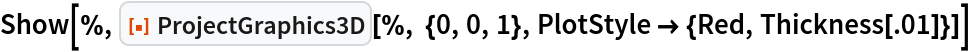Out=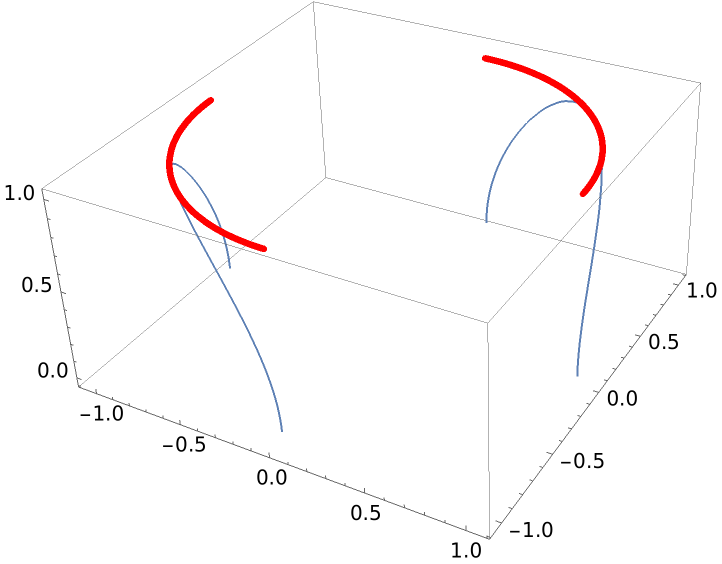Define a graphic using a callout wrapper and show it along with its projection:

 In:=Out=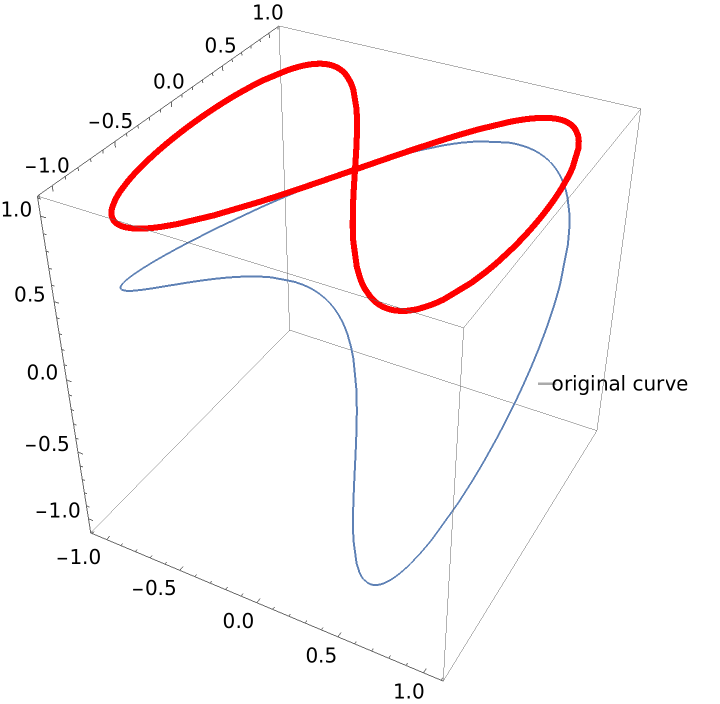Show the correspondence between points in the helix and its projection:

 In:=Out=In:=Out=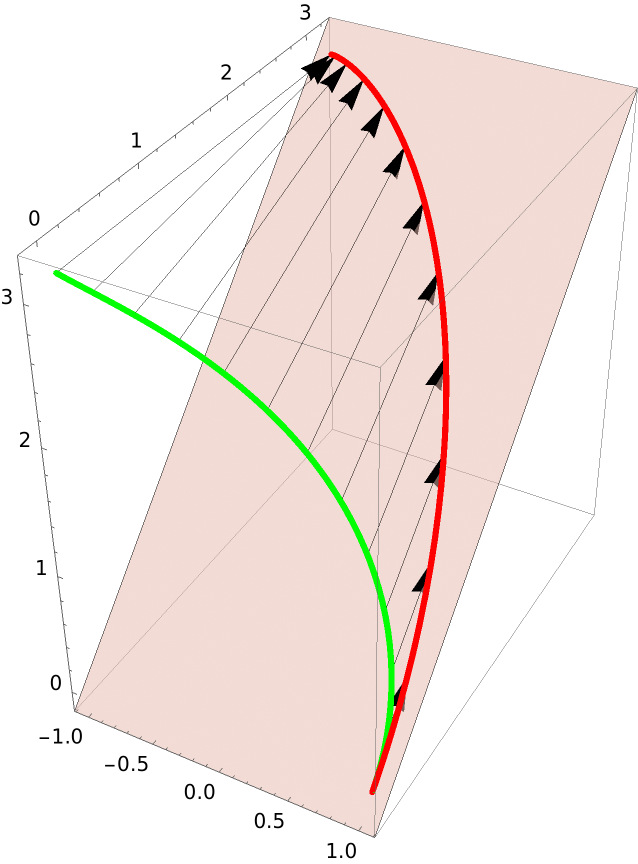Project the curve in orthogonal planes:

 In:=Out=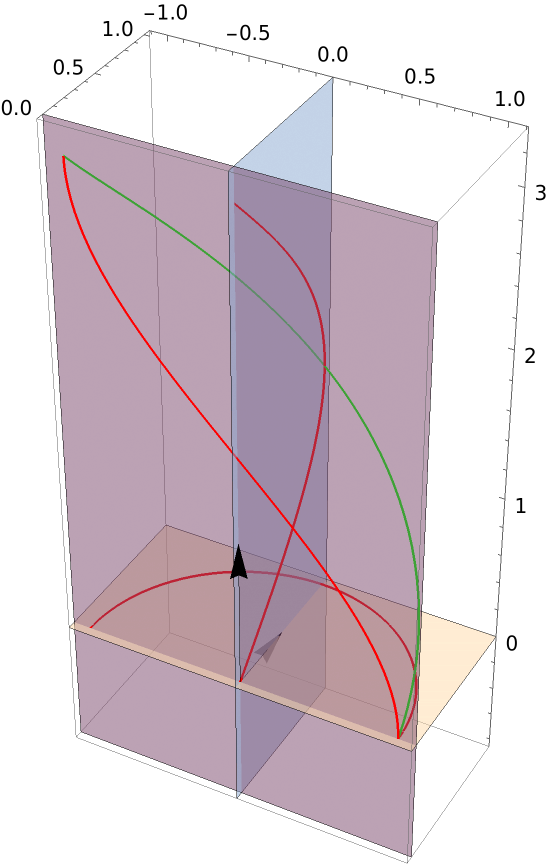The rectifying plane:

 In:=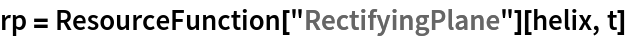Out=The normal plane:

 In:=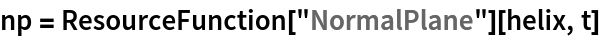Out=The osculating plane:

 In:=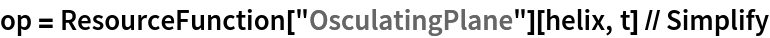Out=Show the projections of the curve in the normal and rectifying planes (the vectors are the tangent, normal and binormal vectors):

 In:=Out=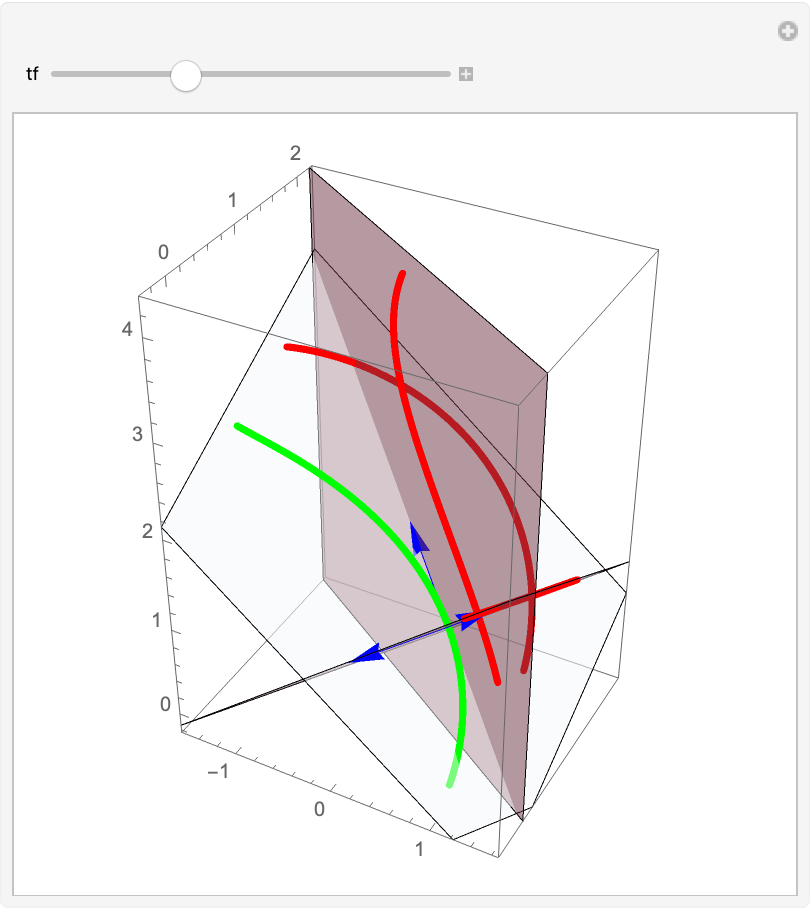### Options (1)

ProjectGraphics3D supports the same options as Graphics3D:

 In:=Out=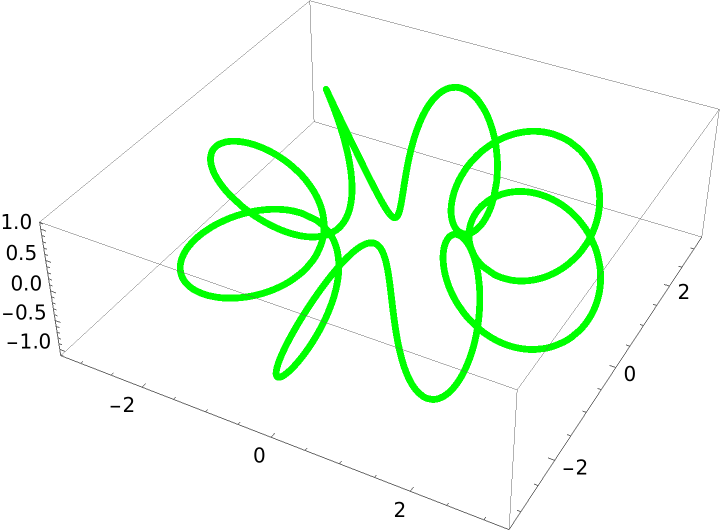In:=Out=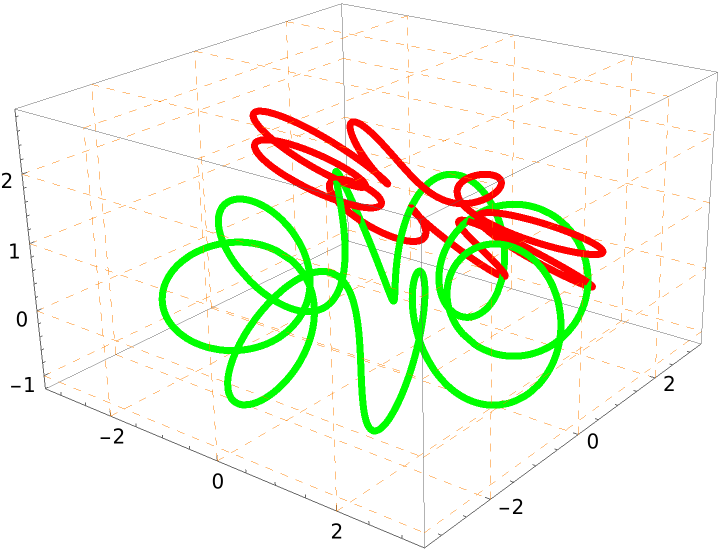### Properties and Relations (3)

Get the equation for a helix:

 In:=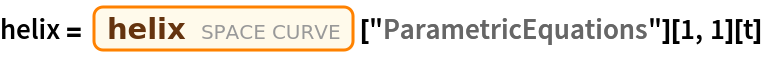Out=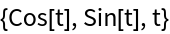Define a graphic output and compute its center:

 In:=Out=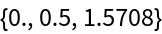Show the curve (green), its projection (red), and the plane of projection:

 In:=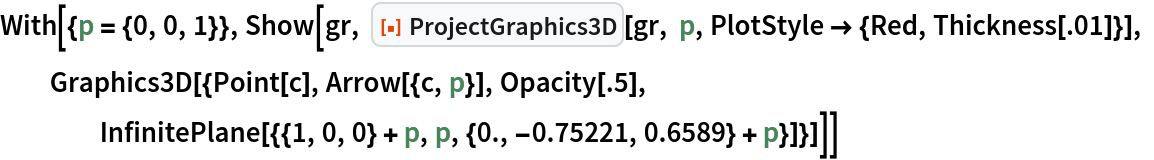Out=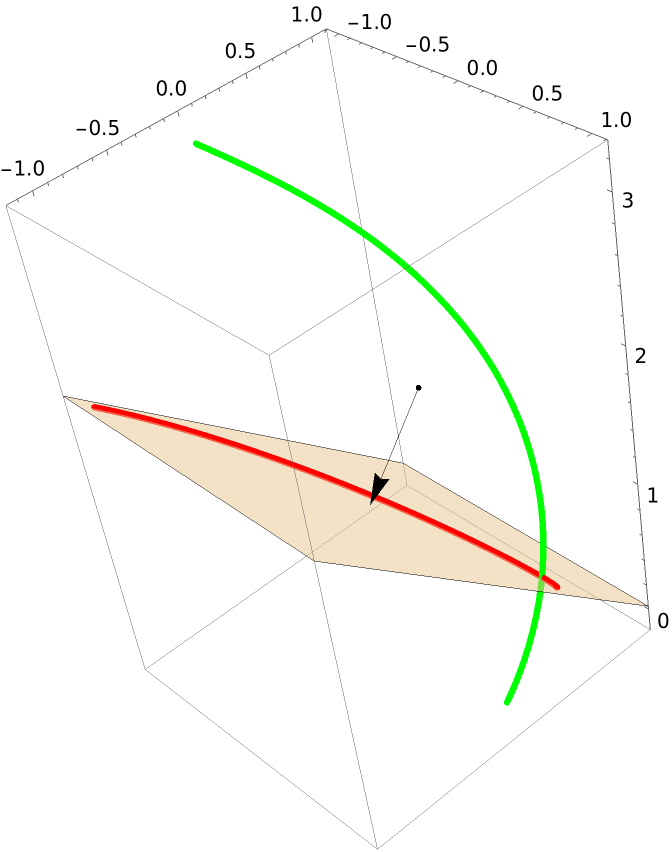### Neat Examples (2)

Hover the mouse over the curve:

 In:=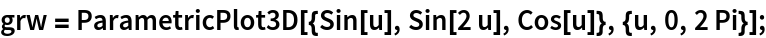In:=Out=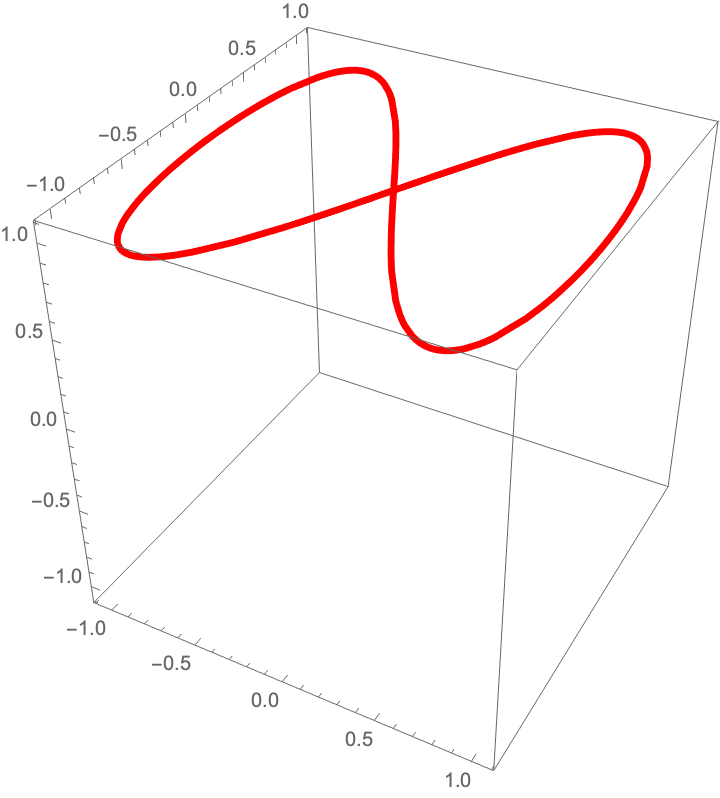Move the spanning vectors and the location point:

 In:=In:=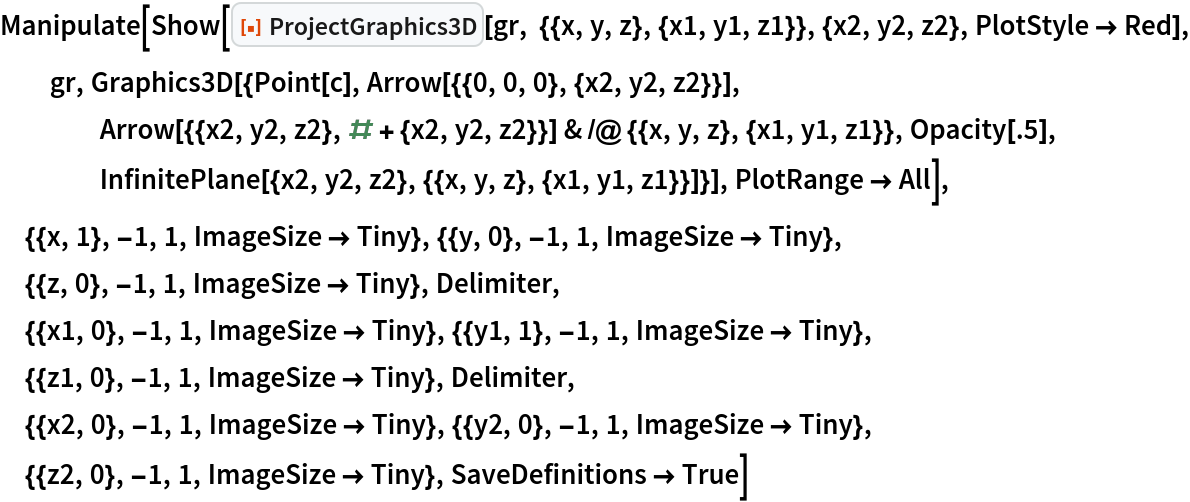Out=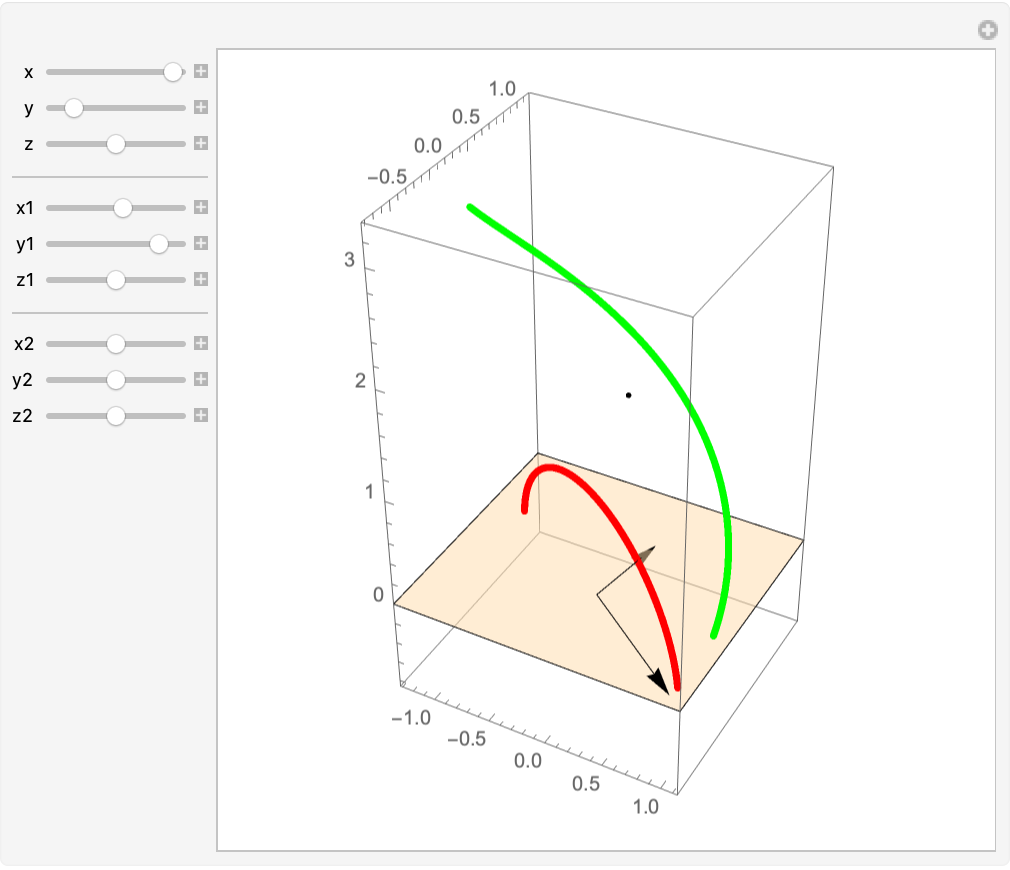Enrique Zeleny

## Version History

• 1.0.0 – 22 April 2021